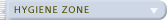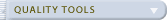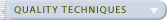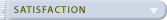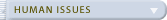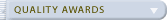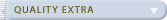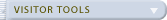Stay Informed Sign up below to receive our Occasional Newsletter.Web SaferPak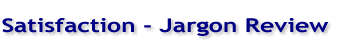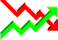Jargon review - glossary of statistical terms and research terms: A B C D E F G H I J K L M N O P Q R S T U V W X Y Z
 Ambiguous questions A question which may confuse respondents, or which they may understand in a different way to that intended. For example, ‘which newspapers do you read regularly?’ – the meaning of the word regularly is unclear. ANOVA (ANalysis Of VAriance) Can be thought of as a generalisation of the t-test to apply to more than two groups. Post-hoc tests can be used to identify where differences are. Attitudinal questions Questions that seek to understand attitudes, motives, values or beliefs of respondents. Behavioural questions Questions that are concerned with what people do, as opposed to what they think. Beta coefficient The weight of a predictor variable in a regression model, indicative of how much impact it has on the outcome variable. Bivariate analysis The analysis of the relationship between two variables – e.g. correlation. Canonical correlation A measure of association between two sets of data, operating through pairs of canonical variates (which can be thought of as similar to factors in factor analysis). Census A survey of the entire population. Chi-Square (?2) Used to test for association between categorical variables. For instance are males more likely to choose to watch sport on television than females? Classification questions Used both for sampling and analysis, they serve as a check that the sample is representative (for example in terms of gender, age and social grade) and also form the basis of breakdown groups for cross-tabulations. Closed questions Questions for which respondents are asked to reply within the constraints of defined response categories. Code of Conduct The MRS Code of Conduct consists of a set of rules and recommendations adhered to by the members of the society. The code prevents research being undertaken for the purpose of selling, and covers issues of client and respondent confidentiality. Coding The process of allocating codes to answers in order to categorise them into logical groups. For example if the question was ‘why are Xyz. the best supplier?’ coding might group answers under ‘Product quality’, ‘Service quality’, ‘Lead times’ etc. Collinearity A data condition that arises when independent variables are strongly related and is a problem when building regression models, leading to unstable beta coefficients. Approaches to counter this problem include factor analysis and ridge regression. Confidence interval The range either side of the sample mean within which we are confident that the population mean will lie. Usually this is reported at the 95% confidence level, in other words we are sure that if we took a 100 similar samples then the mean would fall into this range 95 times. Or more simply, we are 95% sure that the population mean falls in this range. Confirmatory factor analysis A form of factor analysis in which the structure of the data is hypothesised in advance and then tested for goodness-of-fit. Correlation When correlating two variables we measure the strength of the relationship between them. The correlation coefficient is in the range –1 to +1, with the absolute value indicating the strength. A negative coefficient indicates an inverse relationship (i.e. as one goes up the other goes down), 0 indicates no relationship and a positive coefficient indicates a positive relationship. In CSM we would only expect to find positive coefficients. The most common type of correlation is Pearson’s r. Creative comparisons A projective technique in which respondents are asked to liken an organisation to something (frequently a car or an animal) and give reasons, which is what the researcher is interested in. For example: ‘If Xyz was a car, what kind of car would it be? Why?’ – “A Ford Mondeo, because it does its job, but it’s unexceptional, there are lots of others that would do just as well.” CSM Acronym for Customer Satisfaction Measurement. Dependent variable A variable that is assumed to be explained by a number of items (independent variables) also measured. ‘Overall satisfaction’ is the usual dependent variable in CSM. Depth interview A loosely structured, usually face-to-face interview used in exploratory research in business markets, or if the subject matter is considered too sensitive for focus groups. Derived importance Derived importance is based upon the covariation between an outcome variable and a predictor variable. It is usually established by correlation or multiple regression. Desk research Research into secondary data. Diagrammatic scale Also known as a graphic scale, a form of scale without numerical or verbal descriptors but which uses pictures, lines or other visual indicators. Discussion guide The document used by the moderator of a focus group as the equivalent of an interview script, though it is much less structured and prescriptive. Dominance analysis A technique for assessing the relative importance of a series of predictor variables by comparing the average marginal contribution made by each predictor to the model’s R2. Double-barrelled questions Questions which have more than one aspect, for example ‘were the staff friendly and helpful?’ – what if the staff were friendly but not helpful? Endogenous variable See dependent variable. ESM Acronym for Employee Satisfaction Measurement. Exogenous See independent variable. Exploratory research Research undertaken prior to the main survey in order to gain understanding of the subject. In CSM exploratory research should be used to understand what customer requirements are. Face to face interview An interview conducted in person, often at the respondent’s home or office or in the street. Facilitator See moderator. Factor analysis Used to examine relationships in a set of data to identify underlying factors or constructs that explain most of the variation in the original data set. Factors are usually uncorrelated with each other. Factor scores can be calculated and used in order to eliminate the problem of collinearity in data and reduce the number of variables. Feedback Communicating the results of the survey – usually both internally and outside the organisation. Focus group A mainstay of qualitative research, used at the exploratory stage. A group of around 8 people is guided in a discussion of topics of interest by a trained facilitator/moderator. Used for exploratory CSM in consumer markets. Friendly Martian A projective technique in which respondents are asked to advise a friendly alien on the process of interest (say getting a meal at a restaurant), covering all the things he should do, what he should avoid and so on. Since the Martian has no assumed knowledge the respondent will include things that are normally taken for granted. Gap analysis Achieved by subtracting satisfaction scores from importance scores to reveal where satisfaction is most falling short of requirements. Requires interval-level data. Group discussion See focus group. Hypothesis testing Hypothesis testing has a strong tradition in statistics, and is related to confidence interval estimation. A t-test is form of hypothesis test. The procedure is to formulate a null hypothesis (for example that there is no difference between the means of two groups) and then test this and either accept or reject it. Independent variable One of a battery of questions assumed to explain variance in an ‘outcome’ variable such as overall satisfaction – with CSM data these are usually individual requirements such as ‘product quality’. Interval data Numerical scales whose response options are equally spaced, but there is no true zero – e.g. the Celsius scale, the ten-point numerical scale. Item A question on the questionnaire. Kruskal’s relative importance One measure of relative importance. Produces the squared partial correlation averaged over all possible combinations of the predictor variables in a regression equation. Computationally very intensive. Latent Class Regression LCR allows us to identify homogenous subsets in the data that form opinions in the same way, and build separate regression equations for each of these groups. A very young technique that promises to revolutionise the way models are built. Latent variable A variable of interest that cannot be directly measured (for example intelligence) but has to be estimated through procedures such as factor analysis applied to a number of manifest variables deemed to be ‘caused’ by the latent variable (e.g. reading speed, exam results, etc…). Usually form the basis of Structural Equation Models. Leading questions A question that is prone to bias respondents to answer in a particular way, often positively. For example, ‘how satisfied were you…’ as opposed to ‘how satisfied or dissatisfied were you…’. Likert scale A scale running from ‘Strongly agree’ to ‘strongly disagree’ on which respondents rate a number of statements. These should be a combination of positive and negative statements to avoid bias. Linear regression See regression, assumes that the relationship between variables can be summarised by a straight line. Manifest variable A directly measured variable. In procedures such as Confirmatory Factor Analysis and Structural Equation Modelling these are used to construct latent variables. Mean The most common type of average – the sum of scores divided by the total number of scores. Median The central value in a group of ranked data – useful for ordinal-level data. On some occasions the median may be a ‘truer’ reflection of the norm than the mean – for instance average income is usually a median, since the mean is distorted by a few people with very large salaries. Mode The most commonly occurring response. Moderator The researcher leading a focus group. MRS The Market Research Society – the professional body for market researchers in the UK. Implements the Code of Conduct by which most researchers abide and offers Certificate and Diploma qualifications. Multidimensional scaling (MDS) This can be thought of as an alternative to factor analysis. In a similar way it aims to uncover underlying dimensions in the data, but a variety of measures of distance can be used. A common example is to take a matrix of distances between cities (such as that found at the front of a road atlas). Using MDS an analysis in two dimensions would produce something very similar to a map. Multiple regression An extension of simple regression to include the effects of more than one predictor on an outcome variable. Multivariate analysis The analysis of relationships between several variables – e.g. factor analysis. Nominal data Scales that only categorise people, but have no logical ordering – e.g. Male/Female. Non-response bias A major potential source of bias, particularly in postal surveys, in that responders’ opinion may differ from non-responders. For example it is typically those with extreme opinions who respond, or those who feel most involved with your organisation. Normal distribution Graphically represented as a bell curve. Most data has a tendency to fall into this pattern, with people clustering around the mean. The shape of this curve for a variable can be calculated from the mean and standard deviation. The characteristics of the normal distribution are that 68% of scores will be within 1 standard deviation of the mean and 95% will be within 2 standard deviations. This tendency is the basis of assumptions used in confidence interval estimation and hypothesis testing. Numerical scale A scale for which each response option has a numerical descriptor, commonly 1-5, 1-7 or 1-10. The endpoints are usually anchored to provide a direction of response, for example ‘very dissatisfied’ and ‘very satisfied’. Open questions Questions were the respondent’s reply without explicit response categories. These are either coded at the time of interview into existing categories or post-coded. Ordinal data Response categories can be placed in a logical order, but the distance between categories is not equivalent – e.g. Very likely – quite likely – not sure – quite unlikely – very unlikely. Osgood scale See semantic differential scale. Outcome variable See dependent variable. Part correlation See semipartial correlation. Partial correlation The correlation between two numerical variables having accounted for the effects of other variables. This could be used to assess the independent contribution to overall satisfaction of ‘staff friendliness’ having removed a similar variable such as ‘staff helpfulness’. Partial Least Squares (PLS) A technique producing very similar models to Principal Components Regression or Structural Equation Modelling in which the latent variables are constructed in a way that maximises their covariance with the dependent variable. Pilot surveys A survey conducted prior to the main survey using the same instrument, used to assess the questionnaire for potential problems such as respondent confusion or poor routing of questions. Population The group from which a sample is taken, e.g. all of an organisation’s customers for CSM. Postal survey Any survey in which the questionnaire is administered by post. A mail survey in American usage. Post-coding Coding the answers to a question after the survey is complete. Pratt’s relative importance A measure of relative importance that can be thought of as combining a predictor’s total and direct effects on the outcome variable. Calculated as the product of a variable’s correlation and beta coefficient. Pre-coding The process of determining in advance the categories within which respondents’ answers will fall. Predictor variable See independent variable. Primary data Data collected specifically for the question of interest – the CSM survey produces primary data. Principal Components Analysis (PCA) A type of factor analysis. Principal Components Regression (PCR) A form of multiple regression in which the predictor variables are first put through a PCA in order to produce a smaller set of unrelated variables, simplifying the data and eliminating the problem of collinearity. Probability sampling See random sampling. Probing A prompt from the interviewer to encourage more explanation or clarification of an answer. These do not suggest answers or lead respondents but tend to be very general: ‘Anything else’, ‘In what way?’, or even just sounds such as ‘uh-huh’. Projective techniques Common in qualitative research, these are a battery of techniques that aim to overcome barriers of communication based on embarrassment, eagerness to please, giving socially-acceptable answers etc. Examples include theme boards, the ‘Friendly Martian’ and psychodrama. Psychodrama A projective technique also known as role playing. Participants are assigned roles and asked to improvise a short play. Qualitative research Research that aims not at measurement but at understanding. Sample sizes are small and techniques tend to be very loosely structured. Techniques used include focus groups and depth interviews. Quantitative research Research that aims to measure opinion in a statistically valid way, where the limits to the reliability of the measures can be accurately specified. Used at the main survey stage in CSM. Quota sampling A form of non-random sampling in which quotas are set for certain criteria in order to ensure that they are represented in the same proportions in the sample as they are in the population – for example a simple quota might specify a 40%-60% male-female split. R2 The coefficient of determination. This is a measure of how effectively the independent variables in a regression equation predict the outcome variable, for example an R2 of 0.76 suggests that a model accounts for 76% of the variance in the outcome variable. Random sampling Every member of the population has an equal chance of being selected. Ratio data A scale that has a true zero – e.g. the Kelvin scale. You are unlikely to come across this type of data in CSM work. Regression A model that aims to assess how much one variable affects another. This is related to correlation, but implies causality. Requirement A single satisfaction/importance question. Response rate The number of admissible completed interviews, normally represented as a percentage of the number invited to participate. Ridge regression A form of regression analysis that uses a bias parameter (ridge estimator) to alleviate the problem of collinearity. Resulting equations are more stable, but have lower R2 values. Routing Instructions to an interviewer (or respondent in self-completion questionnaires), usually directing them to the next question to be answered based on their previous responses. Sample The people selected from the population to be interviewed. Secondary data Data that already exists, for example government statistics. Self-completion questionnaire A questionnaire that is completed by the respondent rather than by an interviewer. Usually postal surveys, though recent innovations allow Web or email surveys could be used. Semantic differential scale A bipolar diagrammatic scale with opposing adjectives at either end of a series of points (usually seven) on which respondents are asked to mark their opinion. Semipartial correlation The correlation between two variables with the effects of other variables removed from the predictor variable only. SIMALTO scale Acronym for Simultaneous Multi-Attribute Trade-Off. A complex scale that requires respondents to rate their expected, experienced and ideal levels of performance on a variety of key processes. Requires the presence of a skilled interviewer to be reliably completed. Social grade The most common (though now somewhat dated) means of classifying respondents according to socio-economic criteria, based on the occupation of the chief income earner in a household. Classes are A, B, C1, C2, D and E, though these are often grouped into four: AB, C1, C2, DE, or even two: ABC1 and C2DE. Standard deviation The square root of the variance. It can be taken as the average distance that scores are away from the mean. It gives us vital information to reveal the pattern of scores lying behind a mean score. Statistical significance (p-value) This is the confidence we have in confirming or rejecting a hypothesis. For example with a correlation coefficient the significance relates to the confidence we have that the coefficient is not equal to 0. Stratified sampling The population is divided into subgroups of interest and then sampled within these groups. This could be used to ensure that the sample is representative of the relative size/value of the subgroups. Street interview A face-to-face interview conducted in the street or other public place. Structural Equation Modelling (SEM) A close relation of Confirmatory Factor Analysis, this is a powerful technique for hypothesis testing, implemented through specialist software such as LISREL and AMOS. It is a state-of-the-art and very rigorous technique for testing models. Sum The total of all the values for a question. Systematic random sampling Divide the population by the required sample size (e.g. 4000/400 = 10) choose a starting point at random and then select every nth (e.g. 10th) person for interview. Theme board A projective technique involving the use of collages of pictures mounted on card to act as a starting point for a discussion among focus group participants. Pictures might vary from illustrative to metaphorical. t-test Used to test if the difference between the means of two groups is large enough to be significant, in other words that we are confident the difference exists in the population. Unbalanced scale A scale with unequal numbers of positive and negative response categories, leading to a bias in responses. An example is “Excellent” – “Good” – “Average” – “Poor”. Univariate analysis The analysis of a variable on its own – e.g. mean score, variance. Variance A measure of the amount of diversity or variation in the scores received for a question. The analysis of variance is key to many statistical measures of association. Verbal scale Any scale for which answers are given according to a range of phrases or words, as opposed to numerical or diagrammatic scales. The Likert scale is a common example.

 • Satisfaction - ISO 9000:2000 • Satisfaction - Measurement • Satisfaction - Ideas & tips • Satisfaction - FAQ's • Satisfaction - Jargon review • Customer Loyalty

top of pagehome :: about :: contact :: terms © 2006 SaferPak Ltd.